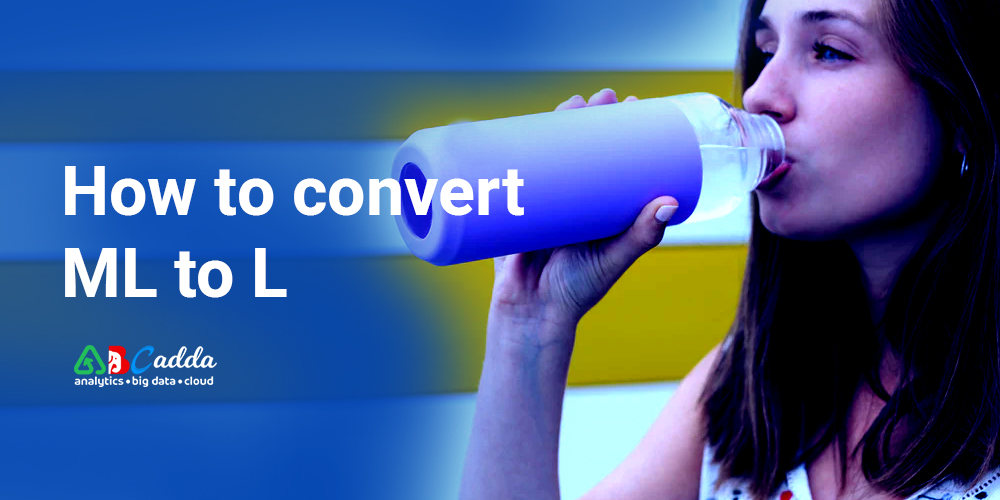# How to convert ml to l

### All-in-one unit converter calculator

Please, choose a physical quantity, two units, then type a value in any of the boxes above.

## Conversion of ml to l using calculator

ml and l are the most common units to measure volume. They are used in the metric system. It is easy to learn the conversion steps between the two measurements. In this blog, we will cover the mL to L conversion.

By the end of this guide, you will get the complete knowledge of mL into L conversions. Keep reading to know more about milliliters to liters.

### What is Millilitres:It is a unit volume that is equal to 1 cubic centimeter or 1/1000 of a liter. It is a SI unit of volume in a metric system. It can be abbreviated as ml. It can also be written as 1 ml, 1 mL.

1 milliliter = 0.001 liters

1milliliter = 1 cubic centimetres

History: the term liter originated from the term “litron”. It was originally part of the French metric system. A milliliter is also referred to as milliliters.

Current use: milliliters used to measure the volume of plastic bottles, cans, juice, milk, toothpaste, etc. The measurement devices such as graduated cylinders, pipette, measurement cups, etc also use the measurement of milliliters.

What is liter: It is a unit volume that is accepted for use with the international system of units. It is equal to 1000 cubic cm.

It is specially defined as the cubic decimeter which is exactly equal to the volume of 1 cubic decimeter. A liter is also referred to as a liter. It can be abbreviated as l.

1 litre = 1000 Millimetres

History: Litre was originally introduced back in 1795 France defined liter as one cubic decimeter. At that time, a liter of water was equal to one kilogram of water.

Current use: it is used to measure liquid volumes such as label containers containing liquids. It is also used to measure non-liquid volumes such as microwaves, refrigerators, computer cases, car trunks.

## Calculation of milliliters present in 1 liter

There are 1000 milliliters in 1 liter. If you want to convert from liters to milliliters, you need to multiply the number by 1000 or divide it by 0.001.

if you want to convert from milliliter then you need to multiply the millimeter value by 0.001 or divide by 1000.

### Millilitres to litres formula:

L = mL/ 1000

For Example, The average water bottle holds 250 mL of water. If you want to pour 14 water bottles into the bucket, how many liters of water would you have?

Step 1: First you need to calculate how many milliliters are there in 20 water bottles.

250 mL × 20 water bottles = 5000 mL

Step 2: convert mL to l by dividing the total number of milliliters in the bucket by 1000. This 1000 is the number of milliliters in a liter.

5000 mL / 1000 mL = 5 litres of water in the bucket.

### What is the use of milliliters calculator:

• This tool can convert metric volume into the desired output.
• This computational calculator is easy and straightforward and provides you with desired conversions in a few simple steps

## How to use millimeters to liters calculator

This calculator is not only used for the conversion of Millilitres to liters. It can be used for a variety of metric and imperial units.

1. To begin the calculation, you need to select the original unit that you want to convert.
2. Enter the number you want to convert in the field.
3. Then you should select the metric unit that you want your unit converted to.

### Applications of mL and L:

Milliliters and liters are used to measure volume. Volume is a measurement of capacity. These two units are used interchangeably to measure appropriate volumes.

Milliliters are used to smaller volumes such as how much milk in a glass. Whereas the liters used to measure larger amounts of volume such as how much water a tank can hold.

## Milliliters to liters conversion

• 0.1 mL = 0.0001 L
• 1 mL = 0.001 L,
• 2 mL = 0.002 L,
• 3 mL = 0.003 L,
• 5 mL = 0.005 L,
• 10 mL = 0.01 L,
• 20 mL = 0.02 L,
• 50 mL = 0.05 L,
• 100 mL = 0.1 L,
• 1000 mL = 1 L,

#### Conclusion

In this blog, you will know the definition of milliliters and liters. You will also know the history between the liters and milliliters and how to convert milliliters into liters. Use our volume calculator for tricky conversions and check all your answers.

Our conversion tool calculator will display the conversion of one unit to another unit in the metric system. Our tool is easy to use and you can get the result instantly. Our unit conversion tool makes the process simpler.

This completes our post about how to convert milliliters to liters. You can find more about liters into milliliters on our page. We appreciate any comments and suggestions you may have at about 1 liter to ml.